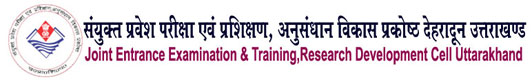Welcome to IRDT Uttarakhand E-Syllabus Web Portal01 CHEMICAL ENGGINEERING
 Duration : 03 Year Eligibility : 10th with Science & Mathematics Intake : 25/40/60(As approved by AICTE/State Govt.) Pattern of the Examination : Semester System Assessment Type : The assessment will we carry out through credit point system/Learning Based Out comes Theory & Practical Ratio : 50: 50 Industrial Training : Included after IInd Semester Ecology & Environment : As pe Government Directions a subject named Environmental Science & Energy Management has been included. Entrepreneurship Development : A subject on Entrepreneurship Development has been incorporated after IInd Semester. General Proficiency : A provision of 6 periods per week has been made. It Included of various cocurricular activities like games, hobby clubs, seminars, declamation contests, extension lectures, NCC, NSS, cultural activities and discipline etc.

### Syllabus

Paper Code :
013002
Paper Name :
FLUID ENGINEERING

#### Classification of fluids:

 Compressible fluids, incompressible fluids, Newtonian and Non-Newtonian fluids, Properties  of  fluids  (only  definitions),  mass  density,  weight  density,  vapour pressure,  specific  gravity,  viscosity,  surface  tension,  compressibility,  thermal conductivity, specific volume.

#### Various types of flow:

 Steady  and  unsteady  flow,  uniform  and  non-uniform  flow,  streamline  flow, laminar and turbulent flow

#### Various types of manometer:

 U-tube  manometer,  inclined  manometer,  differential  manometer  /  two  liquid manometer , simple numerical problems.

#### Basic equations of fluid flow:

 Streamline  and  stream  tube,  Average  velocity,  Mass  velocity,  Equation  of continuity, derivation of the continuity equation, Bernoulli’s theorem, derivation of Bernoulli’s equation, Hagen Poiseulli’s equation, friction factor chart, Fanning equation,  friction  losses  in  pipes,  friction  loss  from  sudden  enlargement  and contraction,  friction  losses  in  various  types  of  fittings  and  valves,  effect  of roughness, Equivalent length, Form friction losses in Bernoulli’s equation, Simple numerical problems related to above topics.

#### Flow measurements:

 Classification of flow meters, principle, construction, working, derivation of flow equation, advantages, disadvantages of (i) Venturimeter (ii) Orifice meter (iii) Pitot tube (iv) Rotameter, difference between Orifice meter and Venturimeter, Measurement of flow in open channels, classification of notches, derivation of total discharge equation of Rectangular notch and V-Notch, advantages of V- Notch over rectangular notch.

#### Transportation of fluids:

 Classification of pipes and fittings, different types of pipes, tubes and fittings,

Schedule number, Birmingham wire gauge (BWG), internal diameter and outer diameter of pipes, the difference between pipe and tube,

Classification of valves, gate valve, globe valve, ball valve, diaphragm valve,

needle valve, butterfly valve,

Classification of pumps,

#### LIST OF PRACTICALS

1.              To determine the co-efficient of discharge of Orifice meter.

2.              To determine the co-efficient of discharge of Venturi meter

3.              To determine the co-efficient of discharge of V-Notches.

4.              To determine the co-efficient of discharge of rectangular notches

5.              To determine coefficient of velocity (Cv). coefficient of discharge (Cd), coefficient of contraction (Cc) and verify the relation between them.

6.              To determine frictional losses in pipes and fittings.

7.              To determine the equivalent length of pipes and fittings.

8.              To verify Bernoulli's Theorem.

9.              To determine the efficiency of a centrifugal pump.

10.           To determine point velocity in a pipe by Pitot tube.

Website Developed By Softmaart